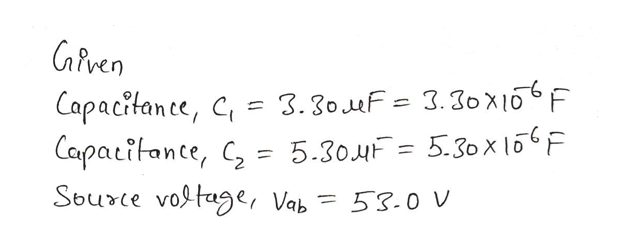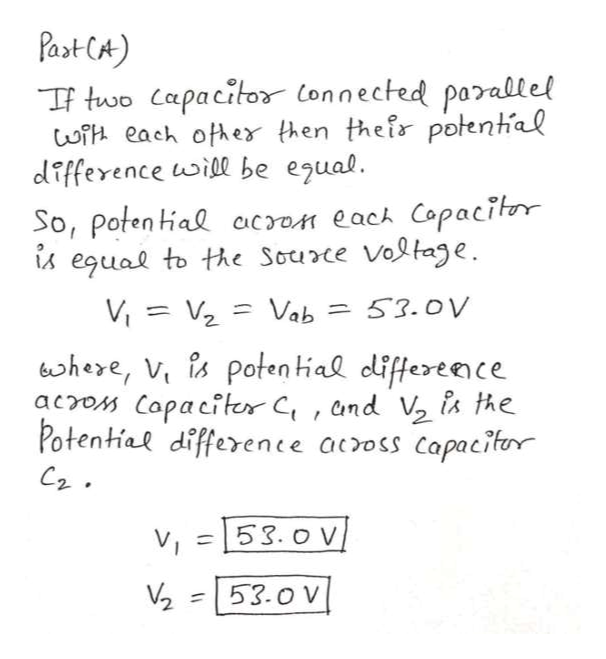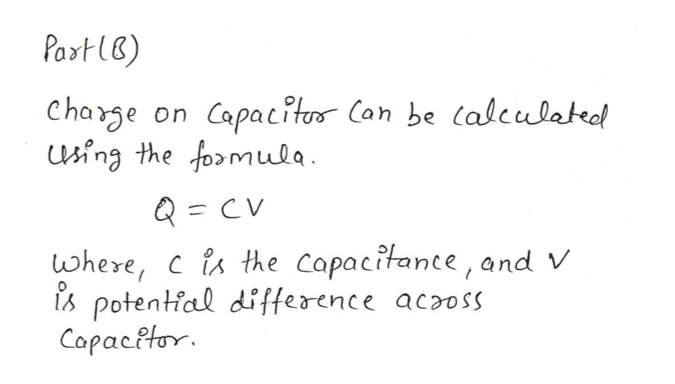# Part A and B PleaseTwo capacitors are connected parallel to each other. Let C 1 = 3.30 μF , C 2 = 5.30 μF be their capacitances, and V ab = 53.0 V the potential difference across the system.  Part A Calculate the potential difference across each capacitor. V 1 and V2 Part B Calculate the charge on each capacitor. Q 1 and Q 2

Question

Two capacitors are connected parallel to each other. Let C 1 = 3.30 μF , C 2 = 5.30 μF be their capacitances, and V ab = 53.0 V the potential difference across the system.

Part A Calculate the potential difference across each capacitor. V 1 and V2

Part B Calculate the charge on each capacitor. Q 1 and Q 2

check_circleExpert Solution
Step 1help_outlineImage TranscriptioncloseCaPven Capacitanee, C 3.30 uF= 3.30X16F Capatitante, = 5.304F = 5.30x l5F Sburce vogtage, Vab 53.0 v fullscreen
Step 2help_outlineImage TranscriptionclosePast CA) TF two Capacitor Connected parallel wi each ofher then their potental difference uwill be egual. So, Poten tial cacron each Copacitor egual to te Source Voltage Vi V Vab 53.0V bhere, V is poten tial differeenc acros Capaciter C, and V is the Potential difference across Capacitor v 53.o V 53.0 V 2 1 fullscreen
Step 3help_outlineImage TranscriptionclosePaxt lB) Charge sing the fomula Capacitu Can be (alculatedl on Q = where, cis the copacitance, and V potential difference acaoss CapacPtor fullscreen

### Want to see the full answer?

See Solution

#### Want to see this answer and more?

Solutions are written by subject experts who are available 24/7. Questions are typically answered within 1 hour*

See Solution
*Response times may vary by subject and question
Tagged in

### Physics